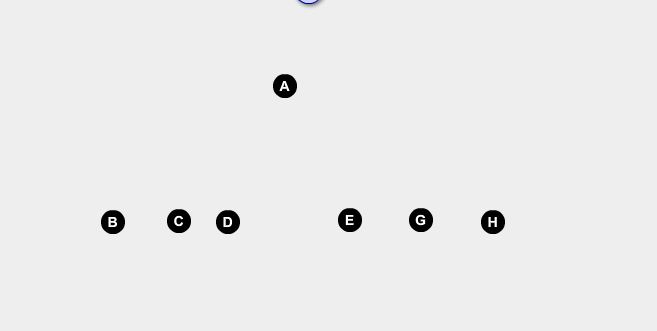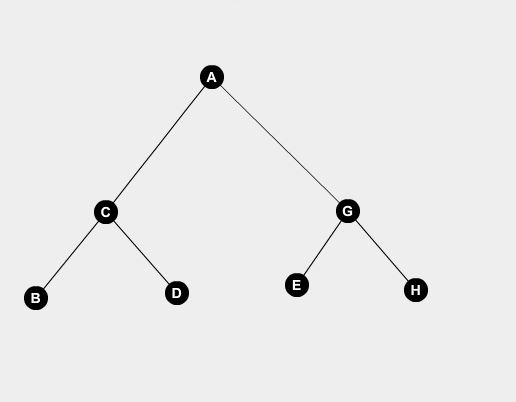# Question & Answer: Given the following inorder and preorder traversals of a binary tree, give the postorder traversal (each letter…..

Given the following inorder and preorder traversals of a binary tree, give the postorder traversal (each letter represents one node). Inorder: BCDAEGH Preorder: ACBDEHG

Don't use plagiarized sources. Get Your Custom Essay on
Question & Answer: Given the following inorder and preorder traversals of a binary tree, give the postorder traversal (each letter…..
GET AN ESSAY WRITTEN FOR YOU FROM AS LOW AS \$13/PAGE

we have
Inorder- BCDAEGH
Preorder- ACBDEHG
we have to calculate postorder-
we know in preorder the leftmost element is the Root element so the root element is
A .
and so from Inorder we can deduct A is the root and BCD is the left elements of A and EGH are the right element of A
so it forms asrecursively we can deduct for subtree BCD C is the root and B is the left of C and D is the right element od C

and for Subtree EGH G is the root and E is left of G and H is the right of G so the tree becomesSo the postorder Traversal is calculated as Left,Right,Root

so the answer is BDCEHGA .